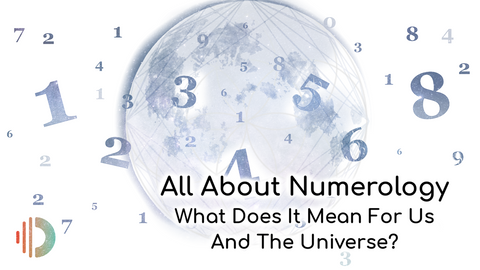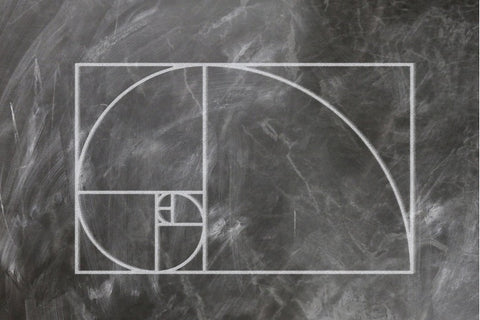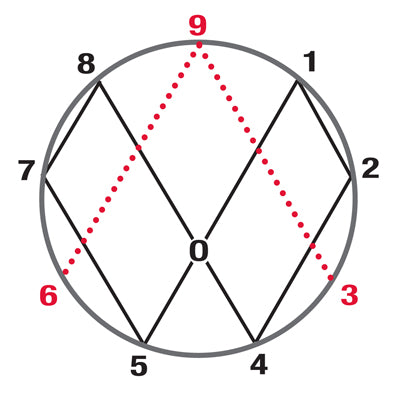# All About Numerology: What Does It Mean For Us And The Universe?

“If you only knew the magnificence of the 3, 6, and 9, then you would have a key to the universe”

• Nikola Tesla
•## What do a spiral galaxy, starfish, human body, and sunflower all have in common?

Not sure? Look deeper.

Their structures are all built upon the same mathematical proportion, called the golden ratio.

This fact seems unbelievable at first glance, almost like we’re reading a made-up fairytale. And yet, here we stand - physicists, biologists, and botanists - all agreeing that some of the biggest and smallest entities in the cosmos originate from the same numeric concept.

Perhaps mathematics is humanity's way of figuring out the divine blueprint that governs all of life.

Numbers weren’t something that we humans invented - rather, we discovered them. If we look closely, numbers form the very fabric of the universe. And even if we remove humans from the equation, you’ll find that the cosmos faithfully follows a set of mathematical equations that govern its past, present, and future.

So is it that hard to believe that numbers can affect our own past, present, and future too?

Here is where the art and science of Numerology comes in, the practice of understanding and unlocking numerical patterns that affect all life and all matter around us. Today let’s delve deeper into the ancient and sacred science of numerology, and what its implications are on our lives, behavior, and destiny.

## History Of Numerology

Even though the growing interest in numerology may seem like a recent phenomenon, it is anything but that. In fact, its foundation was laid by the ancient Babylonians as early as 600 BCE. Later, the Egyptian, Indian, Chinese, and Japanese civilizations also developed their own system of numerology.

However, it is the form of numerology designed by Greek mathematician Pythagoras that forms the foundational basis of western numerology as we know it. Although this brilliant mathematician was famous for his eponymous theorem, he was more interested in the fundamental nature of matter.

Pythagoras’s study revealed to him that all matter in the world is comprised of energetic vibrations, oscillating at certain frequencies. As a result, he developed a system where each letter was had a specific numerical value assigned to it. This gave birth to 'numerology' as we know it.

## What is Numerology?

Before we get into the details of this practice, let’s break down the word itself for clues. ‘Numerology’ is the combination of the Latin word ‘Numerus’ and the Greek word ‘Logos’. While ‘numerus’ signifies numbers, ‘logos’ refers to a ‘word, thought, or expression’. So as you may have already inferred, numerology is the study of numbers, their patterns, and how they reflect in not just our own lives, but in nature and in the cosmos around us.

This ancient study helps us decode the numerical patterns seen everywhere in the universe and in our own lives.

Numerology postulates that our universe is a physical manifestation which is governed by a numerical system - a divine intelligence that governs and brings  order all organic life. In this way, all life 'self-organises' itself according to numerical patterns. Studying numerology helps us interpret and understand this. It can offer us an insight into the fundamental connectedness of everything, be it on an atomic level, on a planetary level or even beyond. The same numerical patterns occur all the way up the harmonic scale.

## How Does Numerology Work?

The key concept used in numerology is that of a ‘digital root’. This is a simple method where we add the digits of a particular number together until the final result only has a single digital remaining.

Let’s take a simple example to explain:

For the number 42, the digital root would be 4+2 = 6

If we look at 412, it would be 4+1+2 = 7

If we take the number 467, then it would be a two-step process to find the digital root:

Step 1: 4+6+7 = 17

Step 2: 1+7 = 8

When applied in the larger context, the digital root of the measurements found in the natural world and geometric figures can reveal hidden patterns in the world around us and the cosmos - unlocking the secret connection between the microcosm and macrocosm.

When applied to humans, taking the digital root of a significant number such as a birth date, birth time, or letters of our name can tell us our life path number, which offers deeper insights into the personality, the past, and the future of that individual.

While calculating the digital root of our birth dates and the letters in our names is fairly easy to do, it takes a qualified and experienced numerologist to fully and accurately decode its true meaning and impact.

## The Link Between Numerology & Sacred Geometry

If you read the example we shared about the golden ratio in the very first sentence of the blog, then you already know the essence of sacred geometry. From celestial bodies to human bodies, all matter carries within it the same pattern of divine intelligence which can be measured. In fact, many call this pattern the ‘Blueprint of God’, because this pattern can be seen everywhere in the universe.

But what does sacred geometry have to do with numerology? As it turns out, a lot!

While numerology studies the fundamental mathematical system that governs the order of the cosmos, then sacred geometry is the visual manifestation, the structure of that divine system.

Sacred geometry gives us visual cues to the divine blueprint that guides the design, flow, and fate of our cosmos. It does so by encoding these numbers, frequencies, and colors into geometric patterns, shapes, and symbols.

### The Fibonacci sequence

One of the best examples of the link between numerology and sacred geometry is demonstrated by the Fibonacci sequence. Named after the medieval Italian mathematician who discovered it, Leonardo Fibonacci, the Fibonacci sequence can be derived this way: every number in the sequence after the first two is the sum of the previous two numbers.

For example, in a Fibonacci sequence:

If the first two numbers are 0 and 1, then the third number will be 0+1 = 1. From hereon, each next number is a sum of the last two,:

0+1 = 1 | 1+1 = 2 | 2+1 = 3 | 3+2 = 5 | 5+3 = 8

And so on, giving us this sequence: 0,1,1,2,3,5,8, 13, 21, 34, 55, 89…..

So what does this sequence look like in its physical (or sacred geometrical) form?Image by Gerd Altmann from Pixabay

And can we see this Fibonacci spiral in nature? Yes! In fact, we can see it everywhere in the universe!Image by Dean Marston from PixabayImage by Hans Braxmeier from PixabayImage by Harald Landsrath from Pixabay

## Numerology, Vortex Math, and Energy

But sacred geometry is not the only phenomenon linked with numerology. The quote we’ve shared by Nikola Tesla gives us a hint into how numerology and vortex math could possibly be connected to uncovering the key to the universe.

Vortex-based mathematics is a dynamic system that explains the relationship of the quality of numbers, rather than their quantity. Discovered by Marko Rodin, vortex math is the science of torus anatomy, and explains the essence of all forms as a sphere within a vortex.

While vortex math deserves its very own blog post, what you need to know at this point is that vortex math reveals that the underlying sequence of energy flow within all matter boils down to the following ever-repeating numerical sequence:

1, 2, 4, 8, 7, 5, and so on (1, 2, 4, 8, 7, 5, 1, 2, 4, 8, 7, 5, 1, 2, 4…).

To explain why, starting at '1':

1

1+1 = 2

2+2 = 4,

4+4 = 8,

8+8 =16, 1+6 = 7

7+7 = 14, 1+4 = 5

5+5 = 10, 1+0 = 1

And this cycle repeats itself to infinity. The beginning is the end is the beginning.In the diagram above, trace this number sequence with our finger:

Go from 1 to 2, to 4 to 8, to 7 to 5, and back to 1

This flow of numbers forms a figure of eight, which represents infinity. This is the flow of the electrical current of the light ring in an atom which continually loops to form matter.

According to Omnia's science, this is because all atoms spin in two phases:

1. The compression of the light ring into matter (represented by the 1, 2, 4, 8, 7, 5, 1 sequence)
2. The discharge of the light ring into the ether (represented by the 3, 6 and 9).

So the etheric field is the invisible energy field that acts as a counterpart to the 'matter' that spins into life as an electrical current in an atom.

If we understand this, it holds many secrets and possibly the key to free energy, something which we know was an obsession of Nikola Tesla.

## Final Thoughts

Every day, we hear of new revelations and discoveries in the world, proving that there is more to the universe than meets the eye. With the tools offered by numerology, sacred geometry, and vortex-based math, we hope that we can progressively come closer to finding out the fundamental connectedness of our lives and everything in the cosmos. Until then, keep an open heart and mind, and never stop pursuing the truth.

https://www.nationalgeographic.org/media/golden-ratio/

https://www.sacredgeometryshop.com/sacred-geometry/sacred-geometry-numerology/

https://eyeofra.blog/2018/12/02/sacred-geometry-numbers-101/